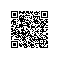# 数组

1 概述 -
2 声明数组变量 -
3 创建数组 -
4 处理数组 -
5 For-Each循环 -
6 数组作为函数的参数 -
7 数组作为函数的返回值 -
8 多维数组 -
9 Arrays类 -

## 1.概述

Java 语言中提供的数组是用来存储固定大小的同类型元素。

## 2.声明数组变量

dataType[] arrayRefVar;   // 首选的方法

dataType arrayRefVar[];  // 效果相同，但不是首选方法

#### 实例

double[] myList;         // 首选的方法

double myList[];         //  效果相同，但不是首选方法

## 3.创建数组

Java语言使用 new 操作符来创建数组，语法如下：

arrayRefVar = new dataType[arraySize];

• 使用 dataType[arraySize] 创建了一个数组。
• 把新创建的数组的引用赋值给变量 arrayRefVar

dataType[] arrayRefVar = new dataType[arraySize];

dataType[] arrayRefVar = {value0, value1, ..., valuek};

#### 实例

public class TestArray {
public static void main(String[] args) {
// 数组大小
int size = 10;
// 定义数组
double[] myList = new double[size];
myList = 5.6;
myList = 4.5;
myList = 3.3;
myList = 13.2;
myList = 4.0;
myList = 34.33;
myList = 34.0;
myList = 45.45;
myList = 99.993;
myList = 11123;
// 计算所有元素的总和
double total = 0;
for (int i = 0; i < size; i++) {
total += myList[i];
}
System.out.println("总和为： " + total);
}
}

总和为： 11367.373## 4.处理数组

#### 示例

public class TestArray {
public static void main(String[] args) {
double[] myList = {1.9, 2.9, 3.4, 3.5};

// 打印所有数组元素
for (int i = 0; i < myList.length; i++) {
System.out.println(myList[i] + " ");
}
// 计算所有元素的总和
double total = 0;
for (int i = 0; i < myList.length; i++) {
total += myList[i];
}
System.out.println("Total is " + total);
// 查找最大元素
double max = myList;
for (int i = 1; i < myList.length; i++) {
if (myList[i] > max) max = myList[i];
}
System.out.println("Max is " + max);
}
}

1.9
2.9
3.4
3.5
Total is 11.7
Max is 3.5

## 5.For-Each循环

JDK 1.5 引进了一种新的循环类型，被称为 For-Each 循环或者加强型循环，它能在不使用下标的情况下遍历数组。

for(type element: array)
{
System.out.println(element);
}

#### 实例

public class TestArray {
public static void main(String[] args) {
double[] myList = {1.9, 2.9, 3.4, 3.5};

// 打印所有数组元素
for (double element: myList) {
System.out.println(element);
}
}
}

1.9
2.9
3.4
3.5

## 6.数组作为函数的参数

public static void printArray(int[] array) {
for (int i = 0; i < array.length; i++) {
System.out.print(array[i] + " ");
}
}

printArray(new int[]{3, 1, 2, 6, 4, 2});

## 7.数组作为函数的返回值

public static int[] reverse(int[] list) {
int[] result = new int[list.length];

for (int i = 0, j = result.length - 1; i < list.length; i++, j--) {
result[j] = list[i];
}
return result;
}

## 8.多维数组

String str[][] = new String;

### 多维数组的动态初始化（以二维数组为例）

1. 直接为每一维分配空间，格式如下：
type[][] typeName = new type[typeLength1][typeLength2];

type 可以为基本数据类型和复合数据类型，arraylength1arraylength2 必须为正整数，arraylength1 为行数，arraylength2 为列数。

int a[][] = new int;

1. 从最高维开始，分别为每一维分配空间，例如：
String s[][] = new String[];
s = new String;
s = new String;
s = new String("Good");
s = new String("Luck");
s = new String("to");
s = new String("you");
s = new String("!");

s=new Strings=new String 是为最高维分配引用空间，也就是为最高维限制其能保存数据的最长的长度，然后再为其每个数组元素单独分配空间 s0=new String("Good") 等操作。

### 多维数组的引用（以二维数组为例）

num;

## 9.Arrays类

java.util.Arrays 类能方便地操作数组，它提供的所有方法都是静态的。

• 给数组赋值：通过 fill 方法
• 对数组排序：通过 sort 方法,按升序
• 比较数组：通过 equals 方法比较数组中元素值是否相等
• 查找数组元素：通过 binarySearch 方法能对排序好的数组进行二分查找法操作

1 public static int binarySearch(Object[] a, Object key)

2 public static boolean equals(long[] a, long[] a2)

3 public static void fill(int[] a, int val)

4 public static void sort(Object[] a)